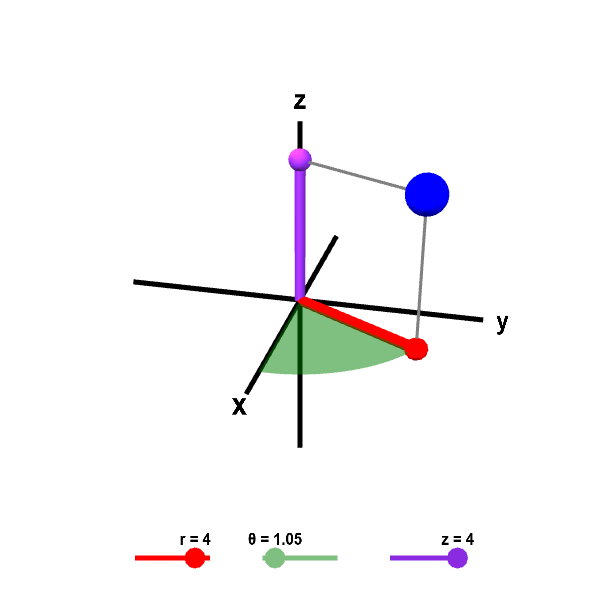# Math Insight

### Applet: Cylindrical coordinatesGiven the values for cylindrical coordinates $r$, $\theta$, and $z$, the large blue point shows the corresponding position in Cartesian coordinates. You can change $r$, $\theta$, and $z$ using the sliders or by dragging the other points shown. The red point is the projection of the large blue point in the $xy$-plane. This projection gives perspective and helps in visualizing $\theta$ and $r$. The coordinate $r$ is the length of the red line segment from the origin to the red point. The coordinate $\theta$ is the angle the red line segment makes with the positive $x$-axis; it is the angle of the green portion of the portion of the disk in the $xy$-plane. The coordinate $z$ is the same as the $z$-coordinate of Cartesian coordinates; it is the height of the purple point on the $z$-axis.